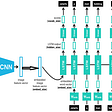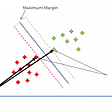# 2D Convolution

For running convolution we need 2 things: input matrix with shape (Batch, Channels, Height, Width) and kernels of our convolution with shape (Out Channels, Input Channels, Height, Width), for each input channel we use different kernel.

Iteratively we get part of the input matrix with the size of kernel and run by element multiplication with each kernel. Sum of element multiplication is our output value. Formula for this A*B = a1*b1 + a2*b2 + …

As we also using strides and input matrix padding we need formula for calculating output matrix shape:
output_w_h = int(((w_h — kernel_w_h + 2 * padding_w_h) / stride_w_h) + 1)

For simplicity we will use square kernels in our example

Of course running such many iteration in real life calculations is not a good idea, so this is just an example to give understanding of logic behind convolution.

# Linear Regression, Ridge Regression and Lasso Regression

Difference between this is only in regularization. Linear regression does not use regularization, Ridge using L1 regularization (lambda*Sum(|Weights|)), Lasso using L2 regularization (lambda*Sum(Weights²))

Optimization process mostly done or by Least Squares method or by Gradient Descent method. I will show the second one as it can be used in many other cases.

So idea of this methods is simple — find the parameters of the line that will pass through the set of points in such way that will minimize out loss function. (For N-dimension points our line will be N-dimension plane)

Formula for the line F(x)= A*X + b
And MSE loss function loss(y_true,y_pred) = Sum((y_true-y_pred)²)/n

So our optimization function for linear regression:
E(x) = loss(y_true,y_pred) = loss(y_true,A*X+b) =
Sum((y_true-A*X-b)²)/n

Now we need to calculate derivative of E(x) by A and b:

dE/dA = (Sum((y_true-A*X-b)²)/n) /dA
By using chain rule we set j = y_true-A*X-b and dE/dA = (dE/dj)*(dj/dA)

dE/dj = (Sum((j)²)/n)dj = 2/n * sum(y_true-A*X-b)
dj/dA = (y_true-A*X-b)/dA = -x

dE/dA = -2/n * x * sum(y_true-A*X-b)

In the same way we getting dE/db = -2/n * sum(y_true-A*X-b)

And now we can use them to calculate update of out weights A and b

For Ridge and Lasso regression we doing the same, except adding L1 and L2 regularization to our optimization forumla

https://gist.github.com/creotiv/9d9911d4707c74d338aa4a4786a48017

# K-means

This algorithm is also pretty simple.
We set number of clusters to N, now set N random centroids(centers of the clusters) and assign nearest points to them forming initial clusters. After this in iteration:
1) for each cluster finding new centroid
2) for each centroids assign points and form new cluster
Iteration stopped when SSE error are not decreasing.

SSE = Sum(euclid(centroid,cluster_points)²), so for each cluster we get sum from squared distances between centroid and cluster points.
For Dimension≤3 we use Euclidian distance

You can run this code in Google Colab

--

--

--

## More from Machine Learning World

The best about Machine Learning, Computer Vision, Deep Learning, Natural language processing and other.

## Recommended from Medium## A separate guide for separable convolutions## Machine Learning Text Classification Project using the Scikit-Learn Library## This Person, Cat, and Horse Does Not Exist## Real-Time Image Captioning on an Embedded System using a light-weight Deep Learning Model (Part-1)## Support Vector Machine (Supervised Machine learning algorithm)## RL — Imitation Learning## Plant Disease Prediction & Get Cure App Using Artificial Intelligence## Andrey Nikishaev

Machine Learning and Computer Vision Researcher. Founder LearnML.Today

## Image Matching with Shopee## How to Classify Different Dialects of English## Art of Choosing Metrics in Supervised Models Part 2## How to tune a Random Forest model